# Introduction to Modeling Plasma Chemistry in COMSOL Multiphysics®

December 8, 2022

Plasma chemistry is of great importance to plasma modeling. It’s through reactions and collisions, for example, that the interactions between different species in a plasma are specified. With this information, source terms and transport coefficients appearing in the species transport equations can be computed. Here, we present the components of a plasma chemistry as well as where and how to obtain relevant data for plasma modeling. We will also discuss approaches to preparing plasma chemistries.

### Plasma Chemistry Components

In low-temperature plasmas with low ionization degrees, the dominant species are neutrals. This means that the electrons and ions are transported in a background of neutral gas (with which they primarily collide). For the plasmas we are interested in modeling, the electrons have much higher energies than all other species in the plasma, with the electron mean energy being of the order of a few electronvolts and the temperature of the background gas ranging from room temperature to about 1000 K.

In many industrial reactors, the plasma is sustained by applying an electric field that is able to accelerate the electrons to energies where ionization can occur. In this context, electrons can be thought of as the primary vehicle for sustaining a discharge, since they gain energy from the electric field and lose it in collisions with the background gas where the reaction products can be neutral excited states, electron, and ions. Heavy species like excited states and ions can also collide, resulting in charge transfer, ionization, and ion–ion recombination. In a plasma reactor, species are transported by diffusion and migration and eventually reach a surface. The interaction with the surface needs to be described. For instance, it’s normal to assume that the electrons are absorbed when reaching a metal surface and that the ions are neutralized to the ground state.

In summary, the main elements of a plasma chemistry are its species and properties, including transport coefficients, electron impact reactions, heavy species reactions, and surface reactions. These are discussed in more detail below.

### Electron Impact Reactions

Electron impact reactions can be categorized as elastic, excitation, ionization, or attachment. The Electron Impact Reaction feature in the COMSOL Multiphysics® simulation software enables you to define these types of reactions. You can see the settings for an ionization reaction of oxygen in the image below.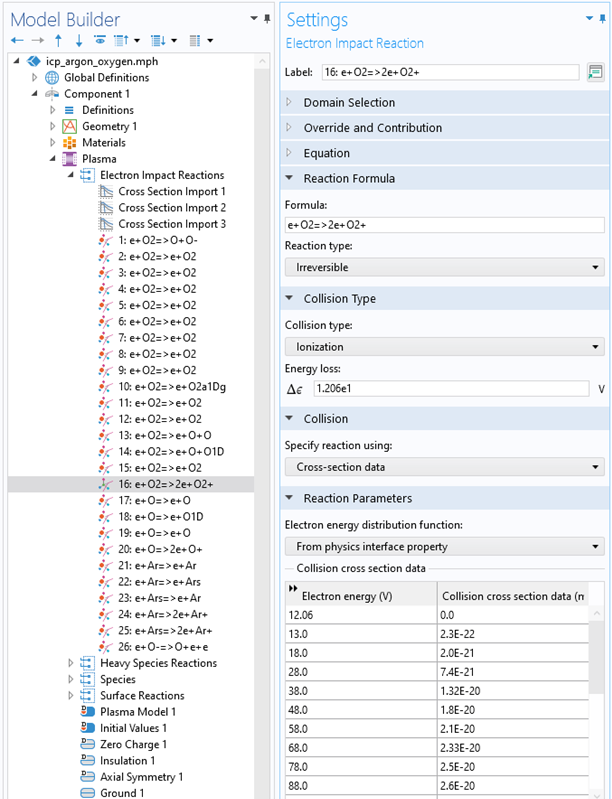Figure 1. The Model Builder showing the Electron Impact Reaction features for a user-made plasma chemistry for a mixture of argon and oxygen. The Settings window is for the Electron Impact Reaction feature that ionizes the molecular oxygen. The reaction is specified by an electron impact cross section.

In elastic collisions, new species are not created, but there is energy transfer between the electrons and neutrals. When an elastic electron impact reaction is set in the Plasma interface, the electron energy loss is accounted for in the electron mean energy equation. Moreover, a heating source term for the background neutral gas is created and can be coupled with the Heat Transfer in Fluids interface. In excitation reactions, an electron can create a new species with higher internal energy. The inverse reaction is also possible, in which case a species de-excites and the electron gains its energy. Ionization reactions are a special case of an excitation, as an electron-ion pair is created. In electron attachment reactions, an electron is captured by a species, and a negative ion is created. This type of reactions tends to be very effective in destroying electrons with low energies.

In the Plasma interface and Plasma, Time Periodic interface, when defining an electron impact reaction, contributions to the source terms of the continuity equations of the species involved are added automatically, and the energy lost or gained by the electrons is added to the source term of the electron mean energy equation.

The source terms in the transport equations are computed using rate coefficients that represent the effect of collisions. For electrons, the best strategy for obtaining rate coefficients is to provide electron impact cross sections and make a suitable integration over the electron energy distribution function (EEDF). The reason for this is that the EEDF is not known a priori, and in low-temperature plasmas, the EEDF often deviates from a Maxwellian EEDF. By providing electron impact cross sections, the flexibility in changing the EEDF is preserved.

In COMSOL®, it’s easy to set an analytic EEDF, import EEDFs, or compute the EEDF using the Boltzmann Equation, Two-Term Approximation interface.

We recommend using the LXCat database to obtain the electron impact cross sections. Reference 1 discusses how to obtain source terms for fluid-type models and how to compute the EEDF.

### Heavy Species Reactions

Understanding the reactions between heavy species can also be important when modeling plasmas. References 2 and 3 are good sources for this topic. Here, only a few reaction types are presented to give an idea of the interactions that can exist between heavy species. The Reaction feature in COMSOL® makes it easy to define heavy species reactions. Figure 2 shows the Settings window where an ion–ion recombination reaction is defined using a rate constant.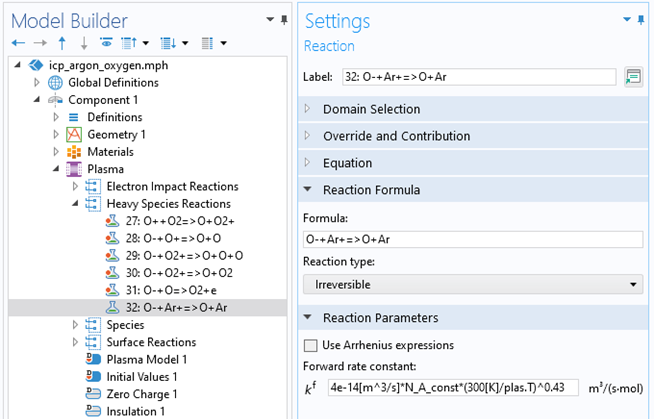Figure 2. The Model Builder showing the Reaction features for a user-made plasma chemistry for a mixture of argon and oxygen. The Settings window is for a Reaction feature that specifies the ion–ion recombination between the oxygen and argon ions.

Penning ionization (A* + A* => e + A+ + A) can occur when the electronic excitation energy of one of the species is larger than the ionization potential of another species. In charge transfer reactions (A+ + B => A + B+), the electron from one neutral species is transferred to a positive ion or from a negative ion to a neutral particle. In ion–ion recombination (A + B+ => A + B), a negative ion and a positive ion interact and produce neutral species. For some operation conditions, this is a negative ion loss mechanism essential for describing electronegative discharges.

Plasma chemistries involving molecular species are more complex because dissociation by electron impact and heavy species association reactions create a very rich system of atomic and molecular species. As an example, in a discharge where the feed gases are SF6, O2, and Ar, new species like F and SO as well as groups like SFx and SOx will also exist.

### Surface Reactions

In COMSOL Multiphysics, surface reactions of heavy species are specified using the Surface Reaction feature, as shown in Figure 3. This feature automatically sets a flux boundary condition for heavy species that is proportional to the species’ thermal velocity.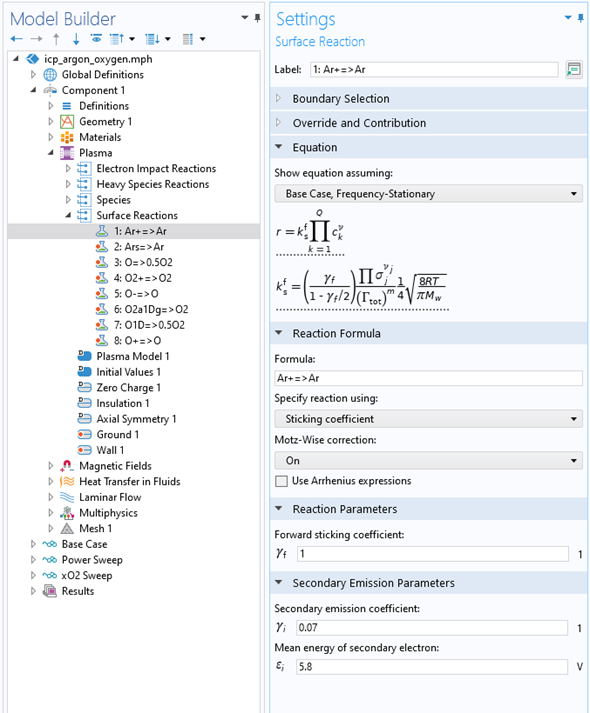Figure 3. The Model Builder showing the Surface Reaction features for a user-made plasma chemistry for a mixture of argon and oxygen. The Settings window is for a Surface Reaction feature that specifies the neutralization of the argon ion at a surface as well as the emission of secondary electrons.

Below, we present some important surface reactions in the perspective of the plasma. The creation and loss of species at surfaces are discussed, but we don’t go into detail about what happens to species at the surface or bulk. (For more information on the latter, try out the Surface Chemistry Tutorial Using the Plasma Module tutorial model.)

#### Losses by Transport

In a plasma reactor, a steady-state operation is achieved by balancing creation mechanisms with volume and surface losses. Frequently, surface losses are the dominant mechanism. In practice, this means that electron impact reactions create an electron-ion pair; the electrons are absorbed at a surface, whereas the ions are neutralized to the ground state. If no loss mechanisms are introduced for a given species (excited states included), the species can grow unbounded. Because of this, a steady state is not possible to achieve, leading to failure of the numerical simulation. Figure 3 shows how the Surface Reaction feature is used to apply a boundary condition for the ion Ar+ and to specify that the ion is neutralized to the ground state Ar at the surface. This is accomplished by typing `Ar+=>Ar` in the Formula field. Similarly, the reaction below ` Ars=>Ar` states that the excited state or argon Ars is de-excited to the ground state.

#### Electron Secondary Emission

When an ion or a neutral excited species reaches a surface, an electron can be emitted. This electron creation mechanism is crucial for the operation of direct current (DC) discharges and to achieve the high-power regime (also known as the gamma regime) in capacitively coupled plasma (CCP) reactors. This mechanism can easily be introduced in COMSOL® by specifying a number different than zero in the Secondary emission coefficient field. In Figure 3, this option is set to 0.07, which means that the ion flux at the surface is multiplied by 0.07, and is given as a flux source to the electrons.

#### Surface Recombination

In molecular discharges, electron impact reactions are very efficient in dissociating a molecule into its constituents. At a surface, recombination can occur. This mechanism can be important in establishing the dissociation degree of the discharge. For the case of oxygen, surface recombination is set by typing `O=>0.5O2` in the Formula field and by specifying the probability of recombination in the Forward sticking coefficient field.

### Transport Coefficients

The fluid-type models used in COMSOL® need transport coefficients for all species in the model. The main transport mechanisms are diffusion and migration in the electric field. These transport mechanisms are characterized by mobility and diffusion coefficients. A good estimation of the transport losses needs accurate transport coefficients. For electrons, the mobility plays an important role in how electrons absorb energy from the electric field.

#### Electron Transport Coefficients

Electron mobility and diffusion coefficients can be computed using electron impact cross sections and knowledge of the EEDF. A common method for describing the EEDF is to solve an approximate form of the Boltzmann equation. In COMSOL Multiphysics, this can be done using the Boltzmann Equation, Two-Term Approximation interface, which is dedicated to solving the Boltzmann equation in the two-term approximation. This can be used to obtain electron transport coefficients and source terms. Reference 1 presents the theory and approximations used in solving the Boltzmann equation in the two-term approximation.

#### Heavy Species Transport Coefficients

For all heavy species, the default setting in the Plasma interface is to compute the diffusion coefficient based on kinetic theory. The equation used to estimate the diffusion coefficient uses the molar mass, potential characteristic length, potential energy minimum, and the dipole moment of each species. (You can learn more about this equation in Reference 4 and in the Species Transport Properties section of the Plasma Module’s User Guide documentation.) This information can be manually introduced or a preset species can be used, as shown in Figure 4. For ions, the species mobility is, by default, computed using the diffusion coefficient and Einstein’s relation. However, you also have the option to specify the mobility and compute the diffusion coefficient using Einstein’s relation. To learn about how ion mobilities can be used as functions of an electric field in a general sense, see Reference 5.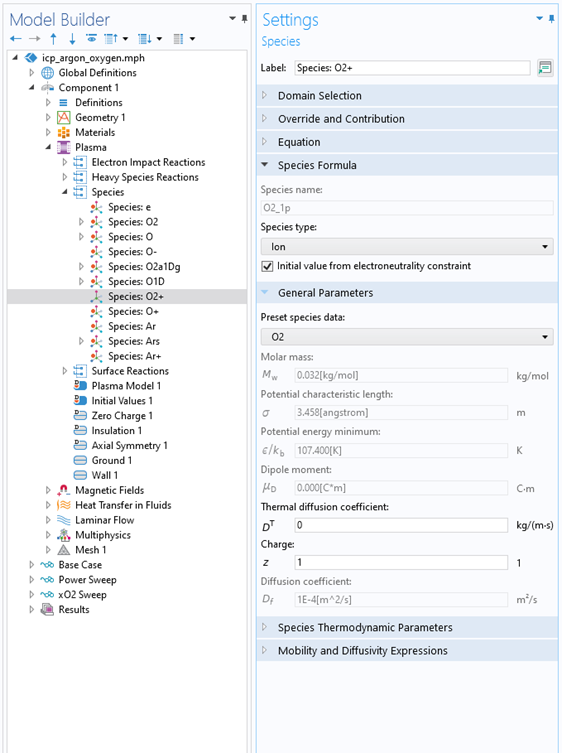Figure 4. The Model Builder showing the Species features for a user-made plasma chemistry for a mixture of argon and oxygen.

### Sources of Data

Plasma chemistry and relevant data can be difficult to obtain, if not inexistent. A great deal of literature research is needed, as is a lot of guess work in many cases. Here, we highlight references that can be used to find data relevant for plasma chemistries. Reference 6, for example, presents how to develop plasma chemistries. The author also gives additional references for plasma chemistry data and discusses how to estimate data. References 2 and 3 are textbooks about plasma physics and plasma chemistry, and provide plasma chemistry data. Reference 5 contains examples of ion mobilities being used as a function of an electric field. To obtain electron impact reactions, we recommend the LXCat database.

The easiest way to obtain a complete plasma chemistry is to find a paper that has already completed the work. An example of this is presented in Reference 7 and Reference 8, where the authors present and discuss plasma chemistries for argon–oxygen mixtures and chlorine plasmas, respectively. The authors use a global model to study the chemistries and use experimental results for validation.

### Workflow for Developing Plasma Chemistries

Plasma chemistry is often used when modeling plasma reactors. However, it’s good practice to separate the preparation of a plasma chemistry from the preparation of a reactor model. When setting up a reactor model, it’s recommended to use a simple plasma chemistry (like the one in the Example 1 section below) to avoid issues related to the plasma chemistry. By doing so, you will be able to focus on other aspects of modeling, such as:

• Investigating how the power is coupled with the system
• Finding a good mesh
• Setting up the couplings with other fluid flow and heat transfer interfaces
• Finding appropriate boundary conditions
• Deciding on a solver strategy

When preparing the plasma chemistry, the first step is to obtain a set of electron impact reactions and, for the reactor’s operating conditions, compute electron transport parameters and source terms using the Boltzmann Equation, Two-Term Approximation interface. This step should make you confident in the cross section set; to help with this, the computed EEDFs, source terms, and transport coefficients should be critically analyzed. The transport parameters, source terms, and EEDFs obtained in this step can be used later in the Plasma interface or Plasma, Time Periodic interface. The Dry Air Boltzmann Analysis model provides a good example of this step.

The next step is to prepare a global model to test and validate the chemistry. In global models, the spatial information of the different quantities in the plasma reactor can be treated as uniform or can be introduced using analytic models. Without the spatial derivatives, the model consists of a set of ordinary differential equations (ODEs). Because of this, the numerical solution becomes considerably simpler and the computational time is reduced. The global model should include the previously tested cross sections as well as all the other components of the plasma chemistry; a good example of how to add chemistry for a global model is the Chlorine Discharge Global Model.

For very large chemistries, it’s better to add reactions and species in small steps and verify that the model still solves. When adding chemistry elements in steps, you might need to adjust the initial conditions and operating conditions to obtain convergence. It’s also important to add the new elements in coherent groups. A few examples are:

1. If a dissociative reaction is added, such as e + O2 => e + O + O, it’s important to also add volume and surface recombination reactions.
2. If a new excited state Ar* is created by the reaction e + Ar => e + Ar*, add surface and volume losses for Ar* and investigate if stepwise ionization is important.
3. If negative ions are created, add volume reactions to destroy negative ions, as losses by transport are often negligible.

In electron impact reactions, the products can be excited states, but solving for the excited states explicitly may not be needed. Reducing the amount of excited states solved for in space-dependent models is a common strategy, and can be tested in a global model first. In COMSOL®, if a reaction like e + N2 => e + N2(A3) is added in the Plasma interface, a species N2(A3) is automatically added together with its transport equation. So, if the excited state does not need to be treated explicitly, you can simply change the reaction formula to e + N2 => e + N2. This way, the electrons still lose energy and change momentum as specified by the cross section, but no excited state is created. Alternatively, you could lump several excited states together and represent them with a generic state N2*. To do this, the reaction e + N2 => e + N2(A3) and the reactions that create similar states are changed to e + N2 => e + N2*.

For the final step, the plasma chemistry that was tested and verified using the global model should be added to the space-dependent model. This step is important, as even if the chemistry was already tested, the space-dependent model contains phenomena related to species transport that were not accounted for in the tests, and they could cause the model to fail. For example, in electronegative discharges, the negative ion density tends to have very sharp transition regions that are difficult to solve for. You can learn about the important aspects and strategies for modeling electronegative discharges with the Model of an Argon/Oxygen Inductively Coupled Plasma Reactor and Model of an Argon/Oxygen Capacitively Coupled Plasma Reactor tutorial models.

### Plasma Chemistry Examples

Before preparing a plasma chemistry, it’s important to establish what you want to learn from the model. If you are working with a model where large tolerances are accepted and you want to estimate the plasma uniformity, power absorption, gas heating, or current at an electrode, a simplified chemistry like the ones presented in the examples below is good starting point. Using such a chemistry is beneficial, as the general idea is to have the plasma chemistry be as simple as possible. However, if you are working with a model that you know requires tighter tolerances, and you wish to learn about specific excited states, a more elaborate chemistry is needed, such as the one presented in Reference 7.

#### Example 1

Figure 5 shows an example of a simple chemistry for an argon plasma implemented in the Plasma interface. A chemistry like this is recommended when preparing the reactor model. It can be found in many of the models in the Application Libraries, such as the DC Glow Discharge model. This chemistry has four species that include electrons, ground state, an effective excited state, and an ion. There are 5 electron impact reactions described by electron impact cross sections: elastic collision with the ground state, excitation to Ars, de-excitation of Ars, ionization from the ground state, and ionization of the excited state. Penning ionization and quenching of the Ars state are included using Reaction features. At the surface, the ions are neutralized and Ars de-excites to the ground state.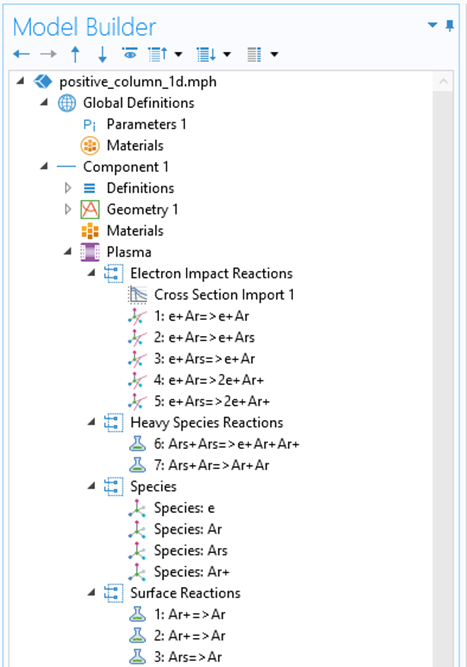Figure 5. Plasma chemistry for argon.

#### Example 2

Figure 6 shows a chemistry for a plasma in a mixture of argon and oxygen. This chemistry is used in the Model of an Argon/Oxygen Inductively Coupled Plasma Reactor and Model of an Argon/Oxygen Capacitively Coupled Plasma Reactor tutorial models and was created to provide a base chemistry for an argon–oxygen mixture, to which more reactions can be added. The argon reactions are the same as in Example 1. Note that the oxygen reactions are based on Reference 2 and Reference 7, and that all of the electron impact cross sections are from LXCat.

Many of the excited states resulting from electron impact reactions are not included in the products to ensure that a new species is not created. (In the Plasma interface, if a new species is added in a reaction, a dependent variable and a transport equation are automatically added.) Only two metastable states of the molecular and atomic oxygen are added, and two reactions are dissociative, resulting in the creation of atomic oxygen. Even if an electron impact reaction does not create new species, the energy lost by electrons is still accounted for in the electron mean energy equation.

Oxygen is an electronegative species, meaning electrons can be attached and create a negative ion (electron impact reaction 1). Even if a surface reaction is included for the negative ion, its losses by transport can be negligible, and the volume losses need to be included (heavy species reactions 28–32).

The dissociation degree is an important aspect of molecular discharges. Naturally, a discharge that is richer in atomic oxygen will be less electronegative since there is less molecular oxygen to attach electrons. In this chemistry, only the surface association mechanisms are included (surface reactions 3 and 7), and they are essential for maintaining realistic dissociation degrees.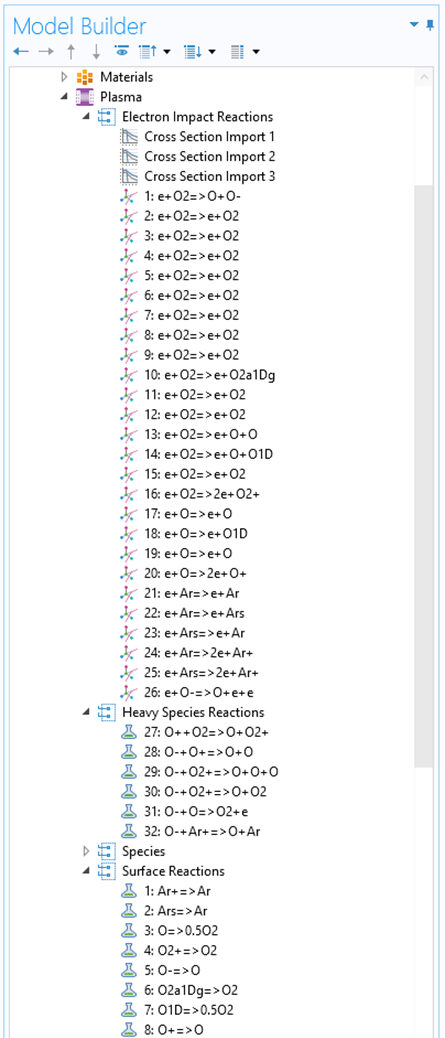Figure 6. Plasma chemistry for a mixture of argon and oxygen.

### Next Step

We encourage you to try setting up plasmas in COMSOL Multiphysics yourself using the various models linked throughout this blog post. If you have any questions about plasma modeling, contact COMSOL via the button below.

### References

1. G.J.M. Hagelaar and L. C. Pitchford, “Solving the Boltzmann equation to obtain electron transport coefficients and rate coefficients for fluid models,” Plasma Sources Science and Technology, vol. 14, pp. 722–733, 2005.
2. M.A. Lieberman and A.J. Lichtenberg, Principles of Plasma Discharges and Materials Processing, John Wiley & Sons, 2005.
3. A. Friedman, Plasma Chemistry, Cambridge University Press, 2008.
4. R.J. Kee, M.E. Coltrin, and P. Glarborg, Chemically Reacting Flow Theory and Practice, Wiley, 2003.
5. H.W. Ellis et al., “Transport properties of gaseous ions over a wide energy range,” Parts I–III, Atomic Data and Nuclear Data Tables, vol. 17, pp. 177–210, 1976; vol. 22, pp. 179–217, 1978; vol. 31, pp. 113–151, 1984.
6. M.J. Kushner, “Strategies for Rapidly Developing Plasma Chemistry Models,” Bull. Am. Phys. Soc., 1999.
7. J.T. Gudmundsson and E.G. Thorsteinsson, “Oxygen discharges diluted with argon: dissociation process,” Plasma Sources Science and Technology, vol. 16, pp. 399–412, 2007.
8. E.G. Thorsteinsson and J.T. Gudmundsson, “A global (volume averaged) model of a chlorine discharge,” Plasma Sources Science and Technology, vol. 19, p. 15, 2010.

#### Categories##### Edgar J. Kaiser
December 21, 2022

Hi Jose,
thanks for this comprehensive blog contribution. Regarding plasma I would be very interested in optical emission. Neutral (or ion) – electron collisions with subsequent photon emission could be seen as a special kind of chemistry. Some time ago I was playing a little with a plasma module test licence, but actually didn’t see a feasible way to calculate optical emission. Do you see a chance in this regard? Could the NIST atomic spectra database some way be used for that? https://physics.nist.gov/PhysRefData/ASD/lines_form.html

Thank you,
Edgar##### Edgar J. Kaiser
January 12, 2023

Any comment?##### Jose Gregorio
January 12, 2023 COMSOL Employee

In the optic of the Plasma interface a reaction between neutral and electron that results in photon emission can be described with an electron impact cross section or a rate constant. The energy lost by the electron is used in the source term of the electron mean energy equation. Spontaneous emission is also possible to include and can be defined using a rate constant. In both cases the radiation in itself is not modeled which means that it leaves the system. It is possible to model radiation transport to some extend using the Radiation in Participating Media interface. If the plasma can be considered optically thick the P1 approximation could be used and the computational cost is low. Keep in mind that there are no predefined couplings between the Plasma and Radiation in Participating Media interfaces, which means the couplings need to be done manually. The general idea is to use the product of the radiation emission reaction set in the Plasma interface as a source term in the radiation transfer equation in the Radiation in Participating Media interface. The resultant radiation flux can be used to compute rate constants for reactions (like excitation and ionization) in the plasma interface.

EXPLORE COMSOL BLOG